• 今天小编就为大家分享一篇Java不指定长度二维数组实例，具有很好的参考价值，希望对大家有所帮助。一起跟随小编过来看看吧
• 展开全部首先说明第一点，你这个代码只是给了一个片段，无法全局的对你编写32313133353236313431303231363533e78988e69d8331333431346430的代码评估并确认问题。现在可以修改的是获取控制台输入的...第步骤：ja...

展开全部

首先说明第一点，你这个代码只是给了一个片段，无法全局的对你编写32313133353236313431303231363533e78988e69d8331333431346430的代码评估并确认问题。现在可以修改的是获取控制台输入的代码可以修改为：

Scanner scanner=new Scanner(System.in);

int n = scanner.nextInt();

第二步骤：java.怎么对长度未知的二维字符数组进行赋值，代码完整编写。

主要使用知识点1：接收控制台输入 Scanner scanner=new Scanner(System.in);

主要知识点2：双层for循环解决二维数组的赋值问题

public static void main(String[] args) {

Scanner scanner=new Scanner(System.in);

System.out.print("请输入一维数组的长度：");

int n = scanner.nextInt();

//换行

System.out.println();

System.out.print("请输入二维数组的长度：");

int m = scanner.nextInt();

//换行

System.out.println();

//创建数组

String[][] strs = new String[n][m];

for (int i = 0; i < strs.length; i++) {

for (int j = 0; j < strs[i].length; j++) {

System.out.print("请输入第"+(i+1)+"个数组的第"+(j+1)+"个元素的值：");

strs[i][j] = scanner.next();

System.out.println();

}

}

System.out.println(strs);

}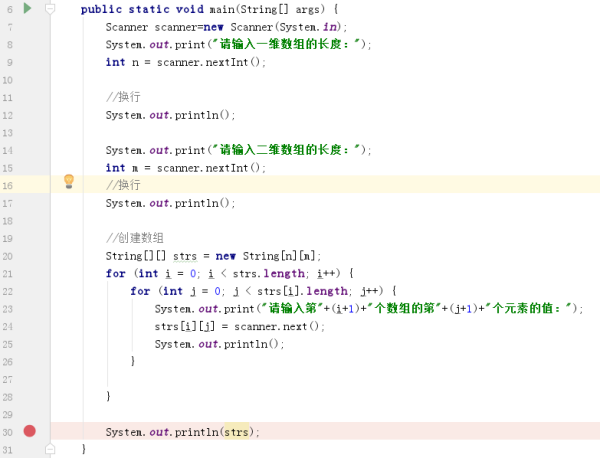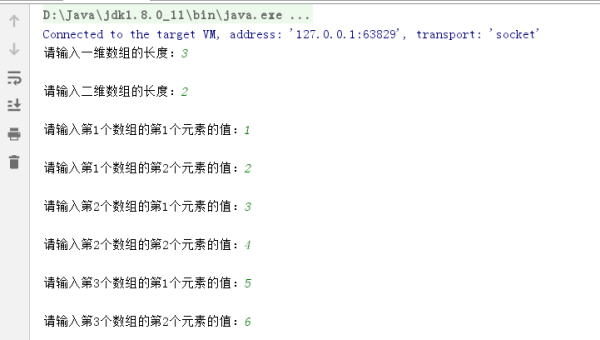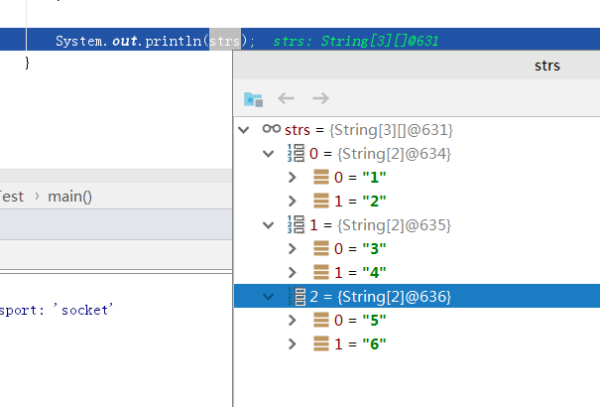展开全文java二维字符数组
• 二维数组，每一维想要输入任意个数的字符用空格隔开时可以用以下代码 import java.util.Scanner; public class Main { public static void main(String[] args) { int a[][]=new int; int n; ...

二维数组，每一维想要输入任意个数的字符用空格隔开时可以用以下代码

import java.util.Scanner;

public class Main {
public static void main(String[] args) {
int a[][]=new int;
int n;
Scanner in=new Scanner(System.in);
n=in.nextInt();
//跳过这行换行符
in.nextLine();
for(int i=0;i<n;i++){
String strLine = in.nextLine();
Scanner s = new Scanner(strLine);
int j=0;
while(s.hasNextInt()){
a[i][j++]=s.nextInt();
}
}
//输出
for( int i=0;i<n;i++){
int j=0;
while(a[i][j]!=0)
System.out.print(a[i][j++]+"  ");
System.out.println();
}

}

}

展开全文• java输入任意长度二维数组

万次阅读 2017-02-18 14:18:51
二维数组，每一维想要输入任意个数的字符用空格隔开时可以用以下代码，如有更好的方法欢迎讨论。 package basic_practice; import java.util.Scanner; public class Main { public static void main(String[] ...

二维数组，每一维想要输入任意个数的字符用空格隔开时可以用以下代码，如有更好的方法欢迎讨论。

package basic_practice;

import java.util.Scanner;

public class Main {
public static void main(String[] args) {
int a[][]=new int;
int n;
Scanner in=new Scanner(System.in);
n=in.nextInt();
//跳过这行换行符
in.nextLine();
for(int i=0;i<n;i++){
String strLine = in.nextLine();
Scanner s = new Scanner(strLine);
int j=0;
while(s.hasNextInt()){
a[i][j++]=s.nextInt();
}
}
//输出
for( int i=0;i<n;i++){
int j=0;
while(a[i][j]!=0)
System.out.print(a[i][j++]+"  ");
System.out.println();
}

}

}

展开全文java 二维数组
• 如何输入固定长度、不定长度二维数组？ 一、如何输入固定长度的一维数组？ 代码实现： import java.util.Scanner; public class Solution { public static void main(String[] args) { //输入一个固定长度的...

Java编程笔试时输入问题：

• 如何输入固定长度、不定长度的一维数组？
• 如何输入固定长度、不定长度的二维数组？
• 如何将数组中的内容直接输出，不要中括号和逗号？

一、如何输入固定长度的一维数组？

• 代码实现：
import java.util.Scanner;

public class Solution {
public static void main(String[] args) {
//输入一个固定长度的一维数组
Scanner in = new Scanner(System.in);
int len = 8;
int[] arr = new int[len];
for(int i = 0; i < len; i++){
arr[i] = in.nextInt();
}
System.out.println(Arrays.toString(arr));
}
}

• 结果显示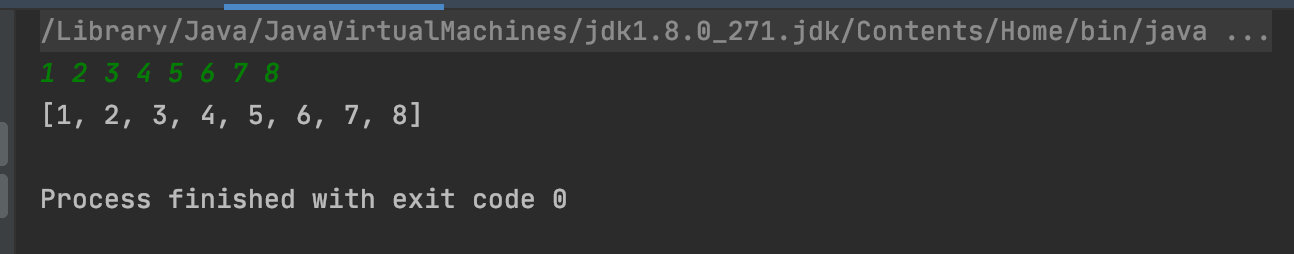二、如何输入不定长度的一维数组？

• 代码实现
import java.util.Scanner;

public class Solution {
public static void main(String[] args) {
//输入一个不定长度的一维数组
Scanner in = new Scanner(System.in);
String s = in.nextLine();
String[] c = s.split(" ");
int[] arr1 = new int[c.length];
for(int i = 0; i < c.length; i++){
arr1[i] = Integer.parseInt(c[i]);
}
System.out.println(Arrays.toString(arr1));
}
}

• 结果显示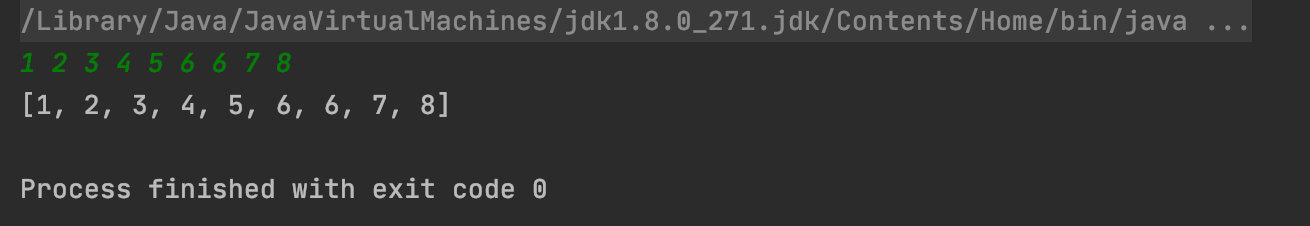三、如何输入固定长度的二维数组？

• 代码实现
import java.util.Scanner;
public class Solution {
public static void main(String[] args) {

//给定一个数组的长m，宽n，然后输出二维数组
Scanner sc = new Scanner(System.in);
int m = sc.nextInt();
int n = sc.nextInt();
int[][] arr = new int[m][n];
for(int i = 0; i < m; i++){
for(int j = 0; j < n; j++){
arr[i][j] = sc.nextInt();
System.out.println(arr[i][j]);
}
}
}
}

• 结果显示
//3和4表示3行4列
3 4
1 2 3 4
1
2
3
4
5 6 7 8
5
6
7
8
3 5 8 0
3
5
8
0

四、如何输入不定长度的二维数组？

• 代码实现
import java.util.*;

public class Solution {
public static void main(String[] args) {
Scanner sc = new Scanner(System.in);
List<int[]> list = new ArrayList<>();
while (sc.hasNextLine()) {
String s = sc.nextLine();
if ("".equals(s)) break;
String[] split = s.split(",");
int[] tem = new int[split.length];
for (int i = 0; i < split.length; i++) {
tem[i] = Integer.valueOf(split[i]);
}
}
}
}

• 结果显示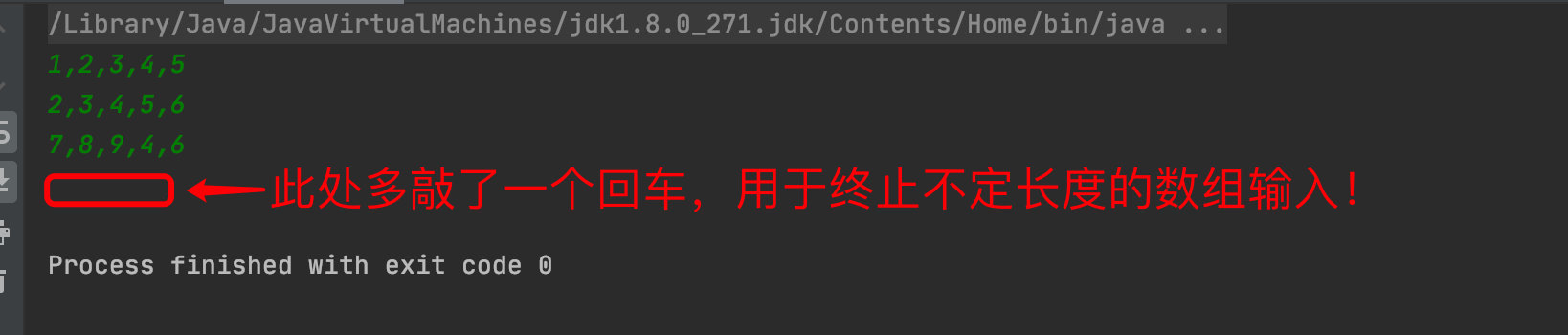五、如何将数组中的内容输出（不要中括号以及逗号）

• 代码实现
int[] arr = new int[]{2,0,2,1,1,0};
for(int i = 0; i < arr.length; i++){
if(i == arr.length - 1){
System.out.print(arr[i]);
}else{
System.out.print(arr[i] + " ");
}
}

• 结果显示展开全文java 数组的输入
• Java不指定长度的二维数组

万次阅读 2016-09-24 21:35:51
编程中经常用到这样一种特殊的二维数组，它的行数确定，但是每行的列数不确定。这样的的数组实现方法：先创建制定行数，列数缺省的二维数组，然后对数组的每一行重新初始化。举个例子就很好理解了。 import java....程序设计 java 二维数组
• Java 读取简单的数字阵列，比如212 33 5512 33 5551 23 5588 955 77981 23 5588 955 77980红色的是输入，黑色的为输出，各个数字之间用空格隔开，开头单独一行表示二维数组的行数，最后的0，表示结束。这个可以用来...
•java 排序算法 算法
• 展开全部importjava.util.Random;publicclassText2{publicstaticvoidmain(String[]args){Matrixs3=newMatrix(4,3);Matrixs4=newMatrix(3,3);s3.printMatrix();System.out.println("------------------...java二维数组相乘
• 前言 首先我们要知道java中数组是要自己分配存储的数量的，所以...public class arrayarray{//二维数组的遍历 public static void main(String args[]){ //定义一个二维数组，不规则 int a[][]={{1,3,4,5},{5...
• 本文知识点(目录)：一、一维数组1、前言如果需要存储大量的数据，例如如果需要读取100个数，那么就需要定义100个变量，显然重复写100次代码，是没有太大意义的。如何解决这个问题，Java语言提供了数组(array)的数据...
• Java数组声明、创建、初始化一维数组的声明方式：type var[]; 或type[] var;声明数组时不能指定其长度(数组中元素的个数)，Java中使用关键字new创建数组对象，格式为：数组名 = new 数组元素的类型 [数组元素的个数]...
• 一、String类String类在java.lang包中，不需要导包，自动导入，字符串变量属于对象。java把String类声明的final类，不能被继承。String类对象创建后不能修改，由0个或多个字符组成，...、String类常用方法1、求...
• 数组的定义 格式1： 元素类型[] 数组名 = new 元素类型[元素个数或数组长度]; 示例：int[] arr = new int; 格式2： 元素类型[] 数组名 = new 元素类型[]{元素，元素，……}; int[] arr = new int[]{3,5,1,7}; ...
• 由于经常在使用矩阵进行计算时，会首先将一维数组转为二维数组。因此，在这里记录一下，也希望对他人有帮助。package deal; /* * author:合肥工业大学 管院学院 钱洋 *1563178220@qq.com */ public class ...数组操作
• 二维数组的使用 二维数组的理解 二维数组是一维数组的数组 内存中并没有存在真正的二维数组，只不过是一维数组中装了一维数组 二维数组的声明和初始化 要想使用二维数组，首先要进行声明和初始化 二维数组的...java
• java中二维数组的定义

千次阅读 2019-09-27 17:38:52
想当于定义了一个3*4的二维数组，数组长度为3，二维数组中每个元素又是一个长度为4的数组 2、int arr[][]=new int[]; 表示定义了一个二维数组arr,里面包含3个数组，每个数组的元素长度不确定 3、int arr[][]={{1,...struts tomcat maven eclipse java
• 声明整型数组的方式(笔者习惯第一种方式，二维数组以第一种方式书写)int[] arr ;int arr1[];2、一维数组的创建Java中使用关键字new创建数组对象，格式为：数组名 = new 数组元素的类型 [数组元素的个数]// 创建数组...
• Java数组之二维数组 Java中除了一维数组外,还有二维数组,三维数组等多维数组.本文以介绍二维数组来了解多维数组. 1.二维数组的基础 二维数组的定义:二维数组就是数组的数组,数组里的元素也是数组. 二维数组表示行列...
• 变长一维数组这里说的变长数组是指在编译时不能确定数组长度，程序在运行时需要动态分配内存空间的数组。实现变长数组最简单的是变长一维数组，你可以这样做：//文件名: array01.cpp#includeusing namespace std;int...
• 看似一个非常简单的问题，但是实际却花了我很多... 首先JAVA中创建二维数组的方法无非两种: 一种是静态的，即已知全部数据，比如要建立3乘3的二维数组，每个数组中的个数，及数组中元素是什么都明确已知，注意，是java 二维数组 实例 arraylist 数据
• 这里不妨定义数组int arr[]，表示n行(n未知)3列的二维数组，我们对它插入元素。 int arr[] = { {1, 2, 3}, {4, 5, 6},{7, 8, 9}, 10 }; 存储顺序示意图如下。 然而它实际在内存中是这样存储的。（理解实际...
• 在学习数组之前，应该先简单了解一下什么数组，这里...一、定义一维数组Type[] name = new Type[number];　//　Type应该是一致的1. int[] arrayList;2. double[] arrayList = new double;3. String[] arrayList ...Java一维数组怎么
• 代表生成一个 一维数组长度是7，一维数组的数组长度未知二维数组 2.静态初始化： int [][] array = new int [][]{  {1,3,5},  {3,5,7,9,1},  {1} } 这里代表生成一个一维数组长度为3，在这个长度为三的一维...
• ArrayList会比Vector快，他是非同步的，如果设计涉及到多线程，还是用Vector比较好一些import java.util.*;public class VectorDemo{public static void main(String[] args){//Vector的创建//使用Vector的构造方法...
• 一、函数（方法） 1、函数的作用： 提高功能代码的复用性 2、函数的定义格式 ...1、二维数组二维数组就是数组中的数组。 2、二维数组的定义格式 数据类型[ ][ ] 变量名 = new 数据类型[长度1...函数 函数的重载 数组
• javascript二维数组转置实例本文实例讲述了javascript二维数组转置的方法。分享给大家供大家参考。具体实现方法如下：代码如下:var arr1 = [[30,-1,90],[70,100,-40],[39,29,6],[39,92,9]];var arr2 = [];//确定新...js 矩阵转置
• 元素类型[] 数组名 = new 元素类型[元素个数或数组长度]; 示例：int[] arr = new int; 格式2： 元素类型[]　数组名 = new 元素类型[]{元素，元素，……}; int[] arr = new int[]{3,5,1,7}; int[] arr = {3,5...Java
• 打怪升级之小白的大数据之旅(七) Java基础语法之数组的排序算法 目录 打怪升级之小白的大数据之旅(七) 上次回顾 ...上一期我们讲完了Java的数组的第一部分，数组的基本概念，本章开始分享...二维数组 进入正题之前..java 编程语言 大数据...

java未知长度二维数组java 订阅